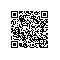public class ConcurrentLinkedQueue<E> extends AbstractQueue<E>
implements Queue<E>, java.io.Serializable


Queue接口中定义的抽象方法有：

package java.util;

public interface Queue<E> extends Collection<E> {

// 向队列中插入元素e，不验证队列空间限制条件下插入一个元素。如果队列有剩余空间，直接插入；如果队列满了，就抛出IllegalStateException异常

boolean offer(E e);

// 返回并删除队列头部的第一个元素，remove()与poll()方法的不同在于，如果队列为空，remove()方法会抛出异常，而poll()方法是返回null
E remove();

// 返回并删除队列头部的第一元素，如果队列空，返回null
E poll();

// 返回但是不删除队头元素，element()方法与peek()方法的不同在于，如果队列为空，element()方法会抛出NoSuchElementException，而peek()方法返回null
E element();

// 返回队头元素，如果队列为空，返回null
E peek();
} 

    private static class Node<E> {
volatile E item;
volatile Node<E> next;
}


    // 头结点，所有后继节点都可以从head开始，使用succ()方法访问到    private transient volatile Node<E> head;
// 尾节点，
private transient volatile Node<E> tail;

头尾节点都提到了succ()方法，succ()方法是

    // sicc()方法是返回节点p的后继节点。如果节点p的后继节点指向自己，则返回头结点。这种情况是如何发生的？（节点p已经不在链表中了？）
final Node<E> succ(Node<E> p) {
Node<E> next = p.next;
return (p == next) ? head : next;
}


succ()方法主要用途有什么？

(1). 求队列大小

    // 返回队列中元素个数，可以看到元素个数是int类型， 如果元素个数超过了Integer.MAX_VALUE的话，也只能返回Integer.MAX_VALUE
// 另外，这个方法返回的值是不精确的。当然我们不是来看size()方法的，是来看succ()方法是如何使用的。
public int size() {
int count = 0;
// 从第一个节点开始遍历，如果节点不为null，统计节点个数，然后使用succ()方法获取下一个节点
for (Node<E> p = first(); p != null; p = succ(p))
if (p.item != null)
// Collection.size() spec says to max out
if (++count == Integer.MAX_VALUE)
break;
return count;
}


(2). contains()方法中succ()的用法与求队列大小类似

    public boolean contains(Object o) {
if (o == null) return false;
for (Node<E> p = first(); p != null; p = succ(p)) {
E item = p.item;
if (item != null && o.equals(item))
return true;
}
return false;
}


    public ConcurrentLinkedQueue() {
head = tail = new Node<E>(null);
}


// 插入元素到队尾
public boolean offer(E e) {
// 检查元素e是否为null，如果为null，抛出NullPointerException
checkNotNull(e);
// 创建新节点newNode
// 首先赋值tail给t (t = tail)，赋值t给p (p = t)
// 然后执行死循环for(;;)
for (java.util.concurrent.ConcurrentLinkedQueue.Node<E> t = tail, p = t;;) {
// 将p的next赋值给q, p.next -> q
// 如果q为null，表示p是尾节点
if (q == null) {
// p是尾节点，将新节点newNode赋值给p的next，p.next -> e(newNode)
// 这个赋值过程是使用CAS来实现的，CAS比较并交换，意思就是如果newNode != null，则交换他们
if (p.casNext(null, newNode)) {
// 如果p != t，即p != t = tail，表示t(= tail)不是尾节点
if (p != t)
// 将t置为尾节点，该操作允许失败，因此t(= tail)并不总是尾节点
// 因此需要执行for(;;)，先找到尾节点
casTail(t, newNode);  // Failure is OK.
return true;
}
}
else if (p == q)
// 如果p == q， 说明尾节点tail已经不在链表中了，
p = (t != (t = tail)) ? t : head;
else
// 如果p == q且q == null，p指向q，即p跳转到下一个元素
p = (p != t && t != (t = tail)) ? t : q;
}
}

public E poll() {
// 跳出for(;;)循环的标志位
for (;;) {
// 然后执行死循环for(;;)
// 获取p的元素值，即头节点的元素值
E item = p.item;
// 如果元素值不为null，并将p的元素置null
// casItem(item, null)意思是如果item != null，则交换两者
// 交换之后，item就从队列中被移除了
if (item != null && p.casItem(item, null)) {
updateHead(h, ((q = p.next) != null) ? q : p);
return item;
}
else if ((q = p.next) == null) { // 说明元素为空
return null;
}
else if (p == q)
else
p = q;
}
}
}


import java.util.concurrent.ConcurrentLinkedQueue;

public class ProducerAndConsumer {

static class Producer extends Thread {
String name;

public Producer(String name) {
this.name = name;
}

public void run() {
for (int i = 0; i < 10; i++) {
queue.offer(i);
System.out.println(name + " : " + i);
try {
} catch (InterruptedException e) {
e.printStackTrace();
}
}
}
}

static class Consumer extends Thread {
String name;

public Consumer(String name) {
this.name = name;
}

public void run() {
for (;;) {
Object item = queue.poll();
System.out.println(name + " : " + item);
try {
} catch (InterruptedException e) {
e.printStackTrace();
}
}
}
}

public static void main(String[] args) {
new Producer("p1").start();
new Producer("p2").start();
new Consumer("c1").start();
// new Consumer("c2").start();
}
}